# WannierBerri advanced tutorial - Fermi surface and Fermi sea calculation of the Berry curvature dipole

author: Jae-Mo Lihm (jaemo.lihm@gmail.com)

The Berry curvature dipole (BCD) is the dipole of the Berry curvature in the reciprocal space. BCD has attracted considerable attention, partially because it contributes to the nonlinear Hall effect.

In WannierBerri, there are two ways to compute this quantity: the Fermi surface integral and the Fermi sea integral:

$D^{\rm surf.}_{ab} = -\int_{\rm BZ} d\mathbf{k} \sum_n \left[ \frac{\partial}{\partial k^a} f_0 (\varepsilon_{n\mathbf{k}}) \right] \Omega^b_{n\mathbf{k}}$
$D^{\rm sea.}_{ab} = \int_{\rm BZ} d\mathbf{k} \sum_n f_0 (\varepsilon_{n\mathbf{k}}) \left(\frac{\partial}{\partial k^a} \Omega^b_{n\mathbf{k}} \right)$

Formally, one can show that the two methods give identical results using partial integration:

$D^{\rm sea.}_{ab} - D^{\rm surf.}_{ab} = \int_{\rm BZ} d\mathbf{k} \frac{\partial}{\partial k^a} \left[ \sum_n f_0 (\varepsilon_{n\mathbf{k}}) \Omega^b_{n\mathbf{k}} \right] = 0.$

The integral is zero because the Brillouin zone has no boundary.

However, in practice, there are numerical differences between the two methods. In this tutorial, we compare the Fermi sea and Fermi surface integral methods for the BCD using the chiral tight-binding model and the ab-initio tight-binding model of ferroelectric GeTe.

:

# Preliminary (Do only once)

# Set environment variables - not mandatory but recommended
import os

import numpy as np
import scipy
import matplotlib.pyplot as plt
import wannierberri as wberri
from wannierberri import calculators as calc

#  This block is needed if you run this cell for a second time
#  because one cannot initiate two parallel environments at a time
try:
parallel.shutdown()
except NameError:
pass

parallel = wberri.Parallel(num_cpus=1, progress_step_percent=10)

2023-08-12 02:17:11,717 INFO worker.py:1519 -- Started a local Ray instance. View the dashboard at 127.0.0.1:8267


## Model 1: Chiral tight-binding model

### Model, band structure

The model is taken from T. Yoda et al, “Orbital Edelstein Effect as a Condensed-Matter Analog of Solenoids”, Nano Lett. 18, 2, 916–920 (2018) https://arxiv.org/abs/1706.07702

The system is a honeycomb lattice with two orbitals per unit cell with an AA stacking (i.e. an AA stacked 2d hexagonal boron nitride). The original model contains in-plance nearest-neighbor hopping (hop1), vertical hopping (hopz_vert), and chiral inter-layer hopping (hopz_left and hopz_right). Here, we additionally add onsite energy difference (delta) and in-plane next-nearest-neighbor hopping (hop2 and phi). Nonzero phi breaks the time-reversal symmetry.

When we set phi=0, the model has time-reversal symmetry and C3z symmetry. In total, there are 6 symmetry operations.

:

import wannierberri.models

# Initiate a tight-binding model (wannierberri.models.Chiral uses PythTB)
model_Chiral_left = wannierberri.models.Chiral(delta=2., hop1=1., hop2=0.3, phi=0, hopz_left=0.2, hopz_right=0.0, hopz_vert=0)

# Initialize WannierBerri system object
system = wberri.System_PythTB(model_Chiral_left, use_wcc_phase=True)

print("=" * 40)

# Set symmetry from the generators.
system.set_symmetry(["TimeReversal", "C3z"])
print("Number of symmetry operations: ", system.symgroup.size)

R=0 found at position(s) []
NOT using ws_dist
Number of wannier functions: 2
Number of R points: 21
Recommended size of FFT grid [3 3 3]
Reading the system from PythTB finished successfully
========================================
Number of symmetry operations:  6


Now, we plot the band structure along a k-point path that passes the high-symmetry k points. We use the plot_path_fat method to plot the band structure.

The energy can be accessed via path_result.get_data("Energy", iband). The valence band maximum is at the Gamma point, while the conduction band minimum on the K-H line.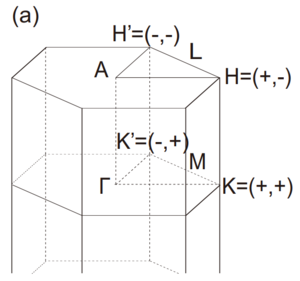Figure taken from T. Yoda et al, Nano Lett. 18, 2, 916–920 (2018).

:

path = wberri.Path(
system,
k_nodes=[
[2/3, 1/3, 0.],  #  K
[0.,  0.,  0.],  #  Gamma
[1/2, 0.,  0.],  #  M
[2/3, 1/3, 0.],  #  K
[2/3, 1/3, 0.5],  #  H
[0.,  0.,  0.5],  #  A
[1/2, 0.,  0.5],  #  L
[2/3, 1/3, 0.5],  #  H
],
labels=["K","$\Gamma$","M","K", "H", "A", "L", "H"],
length=50
)

calculators = {
"tabulate": calc.TabulatorAll(
{"Energy": calc.tabulate.Energy()},
ibands=np.arange(system.num_wann),
mode="path",
)
}

path_result = wberri.run(
system,
grid=path,
calculators=calculators,
parallel=parallel,
print_Kpoints = False,
)

path_result = path_result.results["tabulate"]

calculator not described

Calculation along a path - checking calculators for compatibility
tabulate <wannierberri.calculators.TabulatorAll object at 0x7f304d5baeb0>
All calculators are compatible
Symmetrization switched off for Path
The set of k points is a Path() with 184 points and labels {0: 'K', 33: '$\\Gamma$', 62: 'M', 79: 'K', 104: 'H', 137: 'A', 166: 'L', 183: 'H'}
WARNING : symmetry is not used for a tabulation along path
generating K_list
Done
Done, sum of weights:184.0
symgroup : None
processing 184 K points : using  1 processes.
# K-points calculated  Wall time (sec)  Est. remaining (sec)
18              0.2                   1.8
36              0.2                   0.8
54              0.3                   0.7
72              0.4                   0.6
90              0.5                   0.5
108              0.6                   0.4
126              0.7                   0.3
144              0.7                   0.2
162              0.8                   0.1
180              0.9                   0.0
time for processing    184 K-points on   1 processes:     0.9362 ; per K-point          0.0051 ; proc-sec per K-point          0.0051
time1 =  0.021979331970214844
Totally processed 184 K-points

:

e_vbm = np.amax(path_result.get_data("Energy", 0))
e_cbm = np.amin(path_result.get_data("Energy", 1))
print(f"Valence band maximum   : {e_vbm:6.3f}")
print(f"Conduction band minimum: {e_cbm:6.3f}")

print("VBM k point: ", path_result.kpoints[np.argmax(path_result.get_data("Energy", 0))])
print("CBM k point: ", path_result.kpoints[np.argmin(path_result.get_data("Energy", 1))])

fig = path_result.plot_path_fat(path, close_fig=False, show_fig=False)

ax = fig.get_axes()
ax.axhline(e_vbm, c="k", ls="--")
ax.axhline(e_cbm, c="k", ls="--")
plt.show(fig)

Valence band maximum   : -0.606
Conduction band minimum: -0.099
VBM k point:  [0. 0. 0.]
CBM k point:  [0.66666667 0.33333333 0.16      ]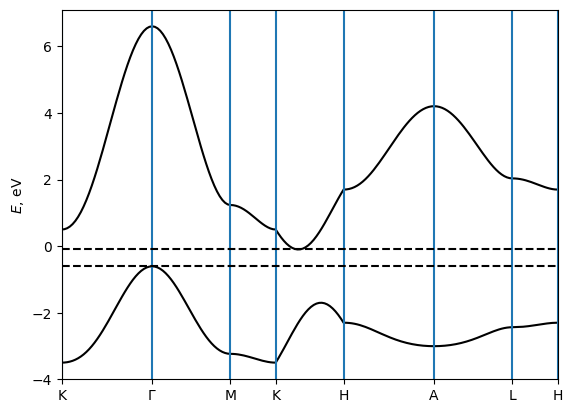### Berry curvature dipole

:

from wannierberri.smoother import FermiDiracSmoother

efermi = np.linspace(-2.0, 1.0, 101, True)
# efermi = np.linspace(e_vbm - 0.5, e_cbm + 0.5, 301, True)

kwargs = dict(
Efermi=efermi,
kwargs_formula={"external_terms": False},
smoother=FermiDiracSmoother(efermi, T_Kelvin=1200, maxdE=8)
)

calculators = {
'berry_dipole_fermi_sea': calc.static.BerryDipole_FermiSea(**kwargs),
'berry_dipole_fermi_surface': calc.static.BerryDipole_FermiSurf(**kwargs),
'dos': calc.static.DOS(Efermi=efermi,),
}

Berry curvature dipole (dimensionless)

| With Fermi sea integral. Eq(29) in Ref <https://www.nature.com/articles/s41524-021-00498-5>_
| Output: :math:D_{\beta\delta} = \int [dk] \partial_\beta \Omega_\delta f

Berry curvature dipole (dimensionless)

| With Fermi surface integral. Eq(8) in Ref <https://journals.aps.org/prl/abstract/10.1103/PhysRevLett.115.216806>_
| Output: :math:D_{\beta\delta} = -\int [dk] v_\beta \Omega_\delta f'

Density of states



Compare calculations using the full k-point grid and the irreducible grid. This can be done using the use_irred_kpt argument of wberri.run.

You can find the following two lines in the output: K_list contains 1000 Irreducible points(100.0%) out of initial 10x10x10=1000 grid K_list contains 172 Irreducible points(17.2%) out of initial 10x10x10=1000 grid

These lines show that using symmetry can speed up calculation by 1000 / 172 ~ 6 times. This speedup corresponds to the 6 symmetry operations. Check that the BCD calculated from the two grids are identical.

:

grid = wberri.Grid(system, NK=20, NKFFT=2)
print("=" * 40)
print("Calculation using the full k-point grid")
result_full_grid = wberri.run(
system,
grid=grid,
calculators=calculators,
parallel=parallel,
print_Kpoints = False,
use_irred_kpt = False,
)

print("=" * 40)
print("Calculation using the irreducible k-point grid")
result_irr_grid = wberri.run(
system,
grid=grid,
calculators=calculators,
parallel=parallel,
print_Kpoints = False,
use_irred_kpt = True,
)

determining grids from NK=20 (<class 'int'>), NKdiv=None (<class 'NoneType'>), NKFFT=2 (<class 'NoneType'>)
The grids were set to NKdiv=[10 10 10], NKFFT=[2 2 2], NKtot=[20 20 20]
========================================
Calculation using the full k-point grid
Grid is regular
The set of k points is a Grid() with NKdiv=[10 10 10], NKFFT=[2 2 2], NKtot=[20 20 20]
generating K_list
Done in 0.02189946174621582 s
Done in 0.02212214469909668 s
K_list contains 1000 Irreducible points(100.0%) out of initial 10x10x10=1000 grid
Done, sum of weights:1.0000000000000007
symgroup : <wannierberri.symmetry.Group object at 0x7f0be010e7f0>
processing 1000 K points : using  1 processes.
# K-points calculated  Wall time (sec)  Est. remaining (sec)
300              3.5                   8.2
600              6.3                   4.2
900              9.0                   1.0
time for processing   1000 K-points on   1 processes:    16.7419 ; per K-point          0.0167 ; proc-sec per K-point          0.0167
time1 =  1.0496785640716553
Totally processed 1000 K-points
========================================
Calculation using the irreducible k-point grid
Grid is regular
The set of k points is a Grid() with NKdiv=[10 10 10], NKFFT=[2 2 2], NKtot=[20 20 20]
generating K_list
Done in 0.015077590942382812 s
excluding symmetry-equivalent K-points from initial grid
Done in 0.11146044731140137 s
Done in 0.11165475845336914 s
K_list contains 172 Irreducible points(17.2%) out of initial 10x10x10=1000 grid
Done, sum of weights:1.0000000000000007
symgroup : <wannierberri.symmetry.Group object at 0x7f0be010e7f0>
processing 172 K points : using  1 processes.
# K-points calculated  Wall time (sec)  Est. remaining (sec)
52              0.8                   1.7
104              1.3                   0.9
156              2.0                   0.2
time for processing    172 K-points on   1 processes:     3.4148 ; per K-point          0.0199 ; proc-sec per K-point          0.0199
time1 =  0.20007848739624023
Totally processed 172 K-points

:

dir_string = ["D_xx", "D_yy", "D_zz"]

fig, axes = plt.subplots(1, 2, figsize=(12, 4))
for label, res in zip(["Full grid", "Irr. grid"], [result_full_grid, result_irr_grid]):
data_fsurf = res.results['berry_dipole_fermi_surface'].data
data_fsea = res.results['berry_dipole_fermi_sea'].data
for i in range(3):
if label == "Full grid":
fmt = f"C{i}-"
else:
fmt = "k--"
axes.plot(efermi, data_fsurf[:, i, i], fmt, label=label + " " + dir_string[i])
axes.plot(efermi, data_fsea[:, i, i], fmt, label=label + " " + dir_string[i])

axes.set_title("Fermi surface")
axes.set_title("Fermi sea")

for ax in axes:
ax.axhline(0, c="k", lw=1)
ax.set_xlabel("Fermi level (eV)")
ax.set_ylabel("Berry curvature dipole")

axes.legend()
plt.tight_layout()
plt.show()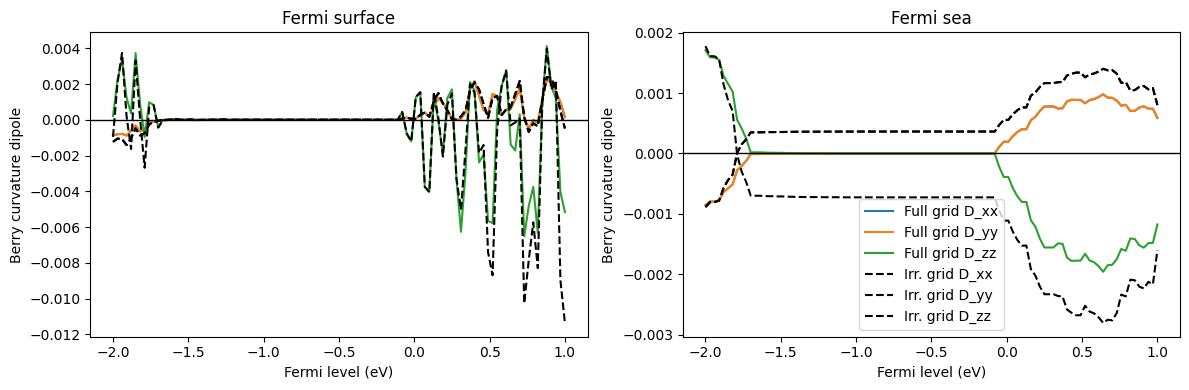From now on, we use the irreducible k-point grid, which is the default in wberri.run.

:

grid = wberri.Grid(system, NK=20, NKFFT=2)
result = wberri.run(
system,
grid=grid,
calculators=calculators,
parallel=parallel,
print_Kpoints = False,
)

determining grids from NK=20 (<class 'int'>), NKdiv=None (<class 'NoneType'>), NKFFT=2 (<class 'NoneType'>)
The grids were set to NKdiv=[10 10 10], NKFFT=[2 2 2], NKtot=[20 20 20]
Grid is regular
The set of k points is a Grid() with NKdiv=[10 10 10], NKFFT=[2 2 2], NKtot=[20 20 20]
generating K_list
Done in 0.018593788146972656 s
excluding symmetry-equivalent K-points from initial grid
Done in 0.11524176597595215 s
Done in 0.11572003364562988 s
K_list contains 172 Irreducible points(17.2%) out of initial 10x10x10=1000 grid
Done, sum of weights:1.0000000000000007
symgroup : <wannierberri.symmetry.Group object at 0x7f0be010e7f0>
processing 172 K points : using  1 processes.
# K-points calculated  Wall time (sec)  Est. remaining (sec)
52              0.6                   1.3
104              1.2                   0.8
156              1.7                   0.2
time for processing    172 K-points on   1 processes:     2.8150 ; per K-point          0.0164 ; proc-sec per K-point          0.0164
time1 =  0.2164595127105713
Totally processed 172 K-points

:

data_fsurf = result.results['berry_dipole_fermi_surface'].data
data_fsea = result.results['berry_dipole_fermi_sea'].data

print("Shape of data: ", data_fsurf.shape)

print("Maximum value for each components (Fermi surface): ")
print(np.linalg.norm(data_fsurf, axis=0))

print("Maximum value for each components (Fermi sea): ")
print(np.linalg.norm(data_fsea, axis=0))

Shape of data:  (101, 3, 3)
Maximum value for each components (Fermi surface):
[[4.79826162e-03 3.60947837e-19 2.68729962e-18]
[5.02055851e-19 4.79826162e-03 5.92648672e-19]
[3.87336309e-19 7.92158054e-20 1.07935885e-02]]
Maximum value for each components (Fermi sea):
[[4.65044414e-03 1.03203601e-19 3.69577356e-18]
[4.43330684e-19 4.65044414e-03 7.09898574e-19]
[3.16277692e-19 6.54682410e-20 9.30088828e-03]]

:

dir_string = ["D_xx", "D_yy", "D_zz"]
for i in range(3):
plt.plot(efermi, data_fsurf[:, i, i], c=f"C{i}", label="Fermi surface " + dir_string[i])
plt.plot(efermi, data_fsea[:, i, i], c=f"C{i}", label="Fermi sea " + dir_string[i], ls="--")

# plt.plot(efermi, result.results['dos'].data, "k-")

plt.axhline(0, c="k", lw=1)
plt.xlabel("Fermi level")
plt.ylabel("Berry curvature dipole")
plt.axvline(e_vbm, c="k", lw=1, ls="--")
plt.axvline(e_cbm, c="k", lw=1, ls="--")
plt.legend()
plt.show()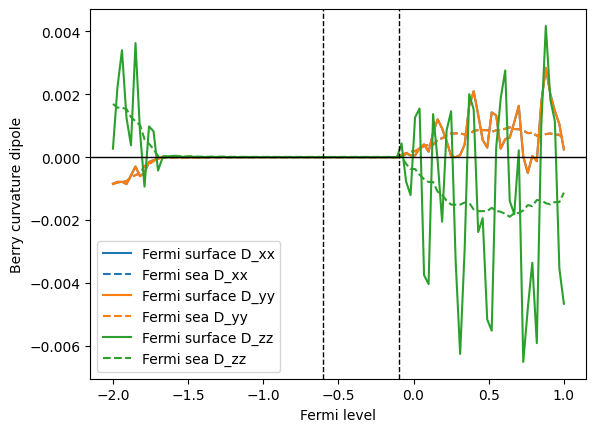The trace of the BCD tensor should be zero due to a topological reason (see footnote 2 of S.Tsirkin et al, PRB 97, 035158 (2018) for details).

The Fermi sea formula for the BCD tensor is explicitly traceless at every k point: this fact can be proven from the definition of the Fermi sea formula using

$\sum_{a} \frac{\partial}{\partial k^a} \Omega^a_{n\mathbf{k}} = \sum_{a} \epsilon_{abc} \frac{\partial}{\partial k^a} \frac{\partial}{\partial k^b} A^c_{n\mathbf{k}} = 0.$

In contrast, tracelessness is not explicit in the Fermi surface formula. The trace of the BCD tensor becomes zero only after summing over the full Brillouin zone due to the cancellation of contributions from all the k points.

Therefore, in numerical calculations using a finite number of k points, only the Fermi sea formula, not the Fermi surface formula, gives a zero trace. The trace will converge to 0 in the Fermi surface case as one makes the k-point grid denser.

:

data_fsurf_trace = np.trace(data_fsurf, axis1=1, axis2=2)
data_fsea_trace = np.trace(data_fsea, axis1=1, axis2=2)

plt.plot(efermi, data_fsurf_trace, label="Fermi surface")
plt.plot(efermi, data_fsea_trace, label="Fermi sea")

plt.axhline(0, c="k", lw=1, zorder=1)
plt.xlabel("Fermi level")
plt.ylabel("Trace(D)")
plt.axvline(e_vbm, c="k", lw=1, ls="--")
plt.axvline(e_cbm, c="k", lw=1, ls="--")
plt.legend()
plt.show()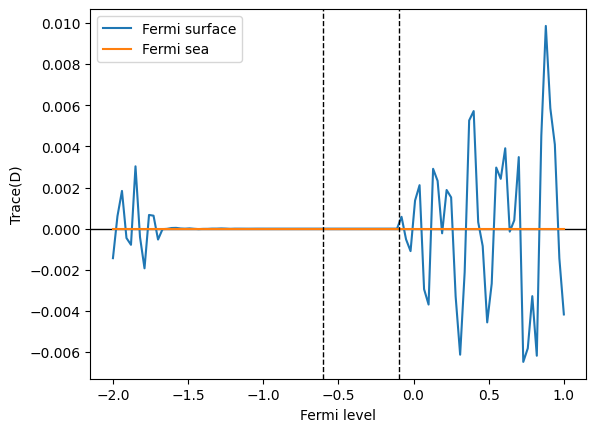Try to calculate the Berry curvature dipole using the two methods for various grid sizes and see how they converge. Also, check that the trace of Berry curvature dipole indeed converges to 0 for both methods.

(NK=120 will be enough to see convergence.)

:

data_fsurf_all = {}
data_fsea_all = {}

:

for nk in [20, 40, 60, 80]:
grid = wberri.Grid(system, NK=nk)
result = wberri.run(
system,
grid=grid,
calculators=calculators,
parallel=parallel,
print_Kpoints = False,
)
data_fsurf_all[nk] = result.results['berry_dipole_fermi_surface'].data
data_fsea_all[nk] = result.results['berry_dipole_fermi_sea'].data

determining grids from NK=120 (<class 'int'>), NKdiv=None (<class 'NoneType'>), NKFFT=None (<class 'NoneType'>)
Minimal symmetric FFT grid :  [3 3 3]
The grids were set to NKdiv=[40 40 40], NKFFT=[3 3 3], NKtot=[120 120 120]
Grid is regular
The set of k points is a Grid() with NKdiv=[40 40 40], NKFFT=[3 3 3], NKtot=[120 120 120]
generating K_list
Done in 1.3817126750946045 s
excluding symmetry-equivalent K-points from initial grid
Done in 6.342855215072632 s
Done in 6.358941316604614 s
K_list contains 10682 Irreducible points(16.69%) out of initial 40x40x40=64000 grid
Done, sum of weights:1.0000000000000344
symgroup : <wannierberri.symmetry.Group object at 0x7f0be010e7f0>
processing 10682 K points : using  1 processes.
# K-points calculated  Wall time (sec)  Est. remaining (sec)
3205             60.7                 141.7
6378            120.8                  81.5
9583            176.0                  20.2
time for processing  10682 K-points on   1 processes:   271.4751 ; per K-point          0.0254 ; proc-sec per K-point          0.0254
time1 =  11.60431694984436
Totally processed 10682 K-points

:

ic = 0
fig, axes = plt.subplots(1, 2, figsize=(12, 4))
nklist = sorted(list(data_fsurf_all.keys()))

for nk in nklist:
c = f"C{ic}"
axes.plot(efermi, data_fsurf_all[nk][:, 0, 0], c=c, label=f"NK={nk}")
axes.plot(efermi, data_fsea_all[nk][:, 0, 0], c=c, label=f"NK={nk}")
ic += 1

axes.set_title("Fermi surface")
axes.set_title("Fermi sea")

for ax in axes:
ax.axhline(0, c="k", lw=1)
ax.set_ylim([-1.5e-3, 1.5e-3])
ax.set_xlabel("Fermi level (eV)")
ax.set_ylabel("Berry curvature dipole")

axes.legend()
plt.tight_layout()
plt.show()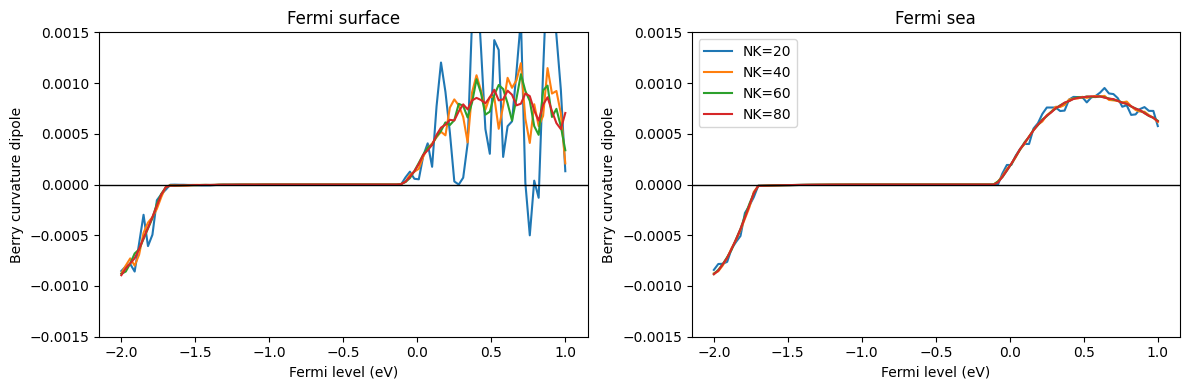:

ic = 0
fig, axes = plt.subplots(1, 2, figsize=(12, 4))
for nk in sorted(list(data_fsurf_all.keys())):
c = f"C{ic}"
axes.plot(efermi, np.trace(data_fsurf_all[nk], axis1=1, axis2=2), c=c, label=f"NK={nk}")
axes.plot(efermi, np.trace(data_fsea_all[nk], axis1=1, axis2=2), c=c, label=f"NK={nk}")
ic += 1

axes.set_title("Fermi surface")
axes.set_title("Fermi sea")

for ax in axes:
ax.axhline(0, c="k", lw=1)
ax.set_ylim([-1.5e-3, 1.5e-3])
ax.set_xlabel("Fermi level (eV)")
ax.set_ylabel("Trace of Berry curvature dipole")

axes.legend()
plt.tight_layout()
plt.show()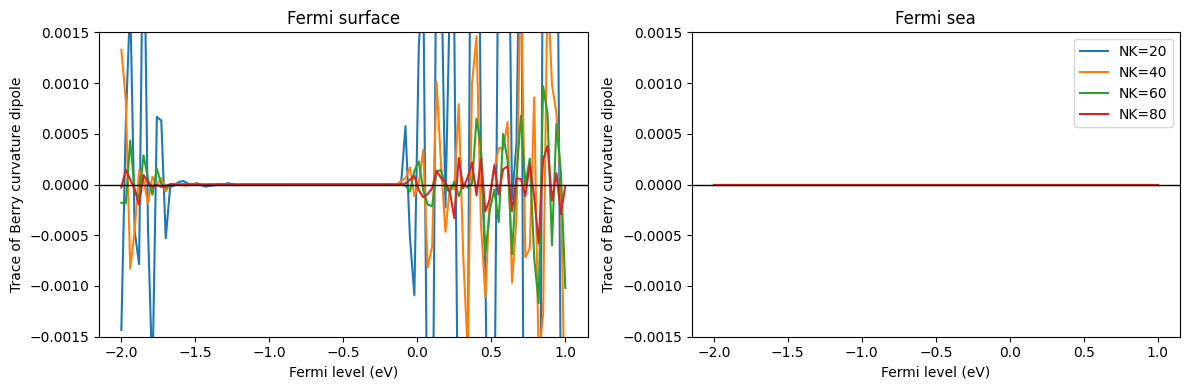:

nklist = sorted(list(data_fsurf_all.keys()))
plt.plot(nklist, [np.linalg.norm(np.trace(data_fsurf_all[nk], axis1=1, axis2=2)) for nk in nklist], "o-", label="Fermi surface")
plt.plot(nklist, [np.linalg.norm(np.trace(data_fsea_all[nk], axis1=1, axis2=2)) for nk in nklist], "o-", label="Fermi sea")
plt.legend()
plt.xlabel("NK")
plt.ylabel("Trace(D)")
# plt.yscale("log")
plt.show()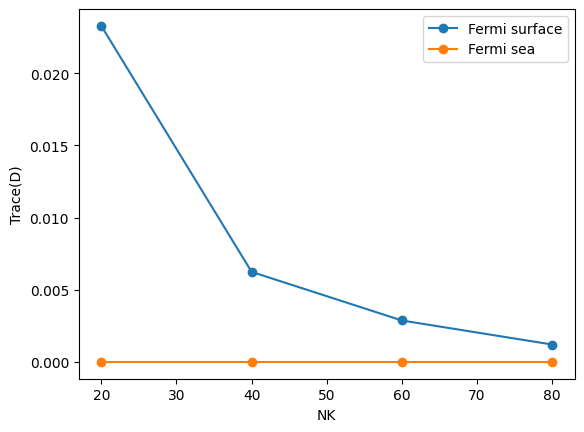## Model 2: Ferroelectric germanium telluride (α-GeTe)

The second model is an ab-initio tighit-binding model of ferroelectric GeTe. This material has a rhombohedrally distorted rocksalt structure where the Te atom is shifted from the center of the unit cell along the  direction.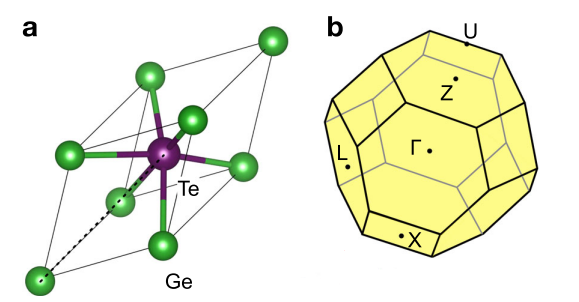Figure taken from H. Wang et al, npj Computational Materials, 6, 7 (2020).

We load the system from a Wannier90 output. We set symmetry using the set_symmetry_from_structure method, which calls spglib to automatically determine the symmetry of the system.

We also symmetrize the system. See data_GeTe/GeTe.win file and check that the initial projections are correct. For details, refer to the symmetrization tutorial.

:

system_GeTe = wberri.System_w90("data_GeTe/GeTe", berry=True)
system_GeTe.set_structure([[0., 0., 0.], [0.4688262167, 0.4688262167, 0.4688262167]], ["Ge", "Te"])
system_GeTe.set_symmetry_from_structure()

system_GeTe.symmetrize(
proj=["Ge:s", "Ge:p", "Te:s", "Te:p"],
atom_name=["Ge", "Te"],
positions=[[0., 0., 0.], [0.4688262167, 0.4688262167, 0.4688262167]],
soc=False,
DFT_code='qe'
)

using fortio to read
Reading restart information from file ../data_GeTe/GeTe.chk :
Time to read .chk : 0.13194584846496582
Time for MMN.__init__() : 0.3212873935699463 , read : 0.31705737113952637 , headstring 0.004230022430419922
time for FFT_q_to_R : 0.010440587997436523 s
using ws_distance
irvec_new_all shape (37,)
using ws_dist for Ham_R
using ws_dist for AA_R
Number of wannier functions: 8
Number of R points: 63
Recommended size of FFT grid [3 3 3]
Real-space lattice:
[[ 2.11676981 -1.22211762  3.64031036]
[ 0.          2.44423524  3.64031036]
[-2.11676981 -1.22211762  3.64031036]]
Wannier atoms info
(1, 'Ge', [0.0, 0.0, 0.0], ['s', 'p'], [, [1, 2, 3]])
(2, 'Te', [0.4688262167, 0.4688262167, 0.4688262167], ['s', 'p'], [, [5, 6, 7]])
[get_spacegroup]
Spacegroup is R3m (160).
---------------    1 ---------------
det =  0.9999999999999999
rotation:                    cart:
[ 1  0  0]                    [1.00 -0.00 -0.00]
[ 0  1  0]                    [-0.00 1.00 -0.00]
[ 0  0  1]                    [-0.00 0.00 1.00]
translation:
( 0.00000  0.00000  0.00000)  ( 0.00000  0.00000  0.00000)
---------------    2 ---------------
det =  1.0
rotation:                    cart:
[ 0  1  0]                    [-0.50 0.87 0.00]
[ 0  0  1]                    [-0.87 -0.50 -0.00]
[ 1  0  0]                    [-0.00 0.00 1.00]
translation:
( 0.00000  0.00000  0.00000)  ( 0.00000  0.00000  0.00000)
---------------    3 ---------------
det =  1.0
rotation:                    cart:
[ 0  0  1]                    [-0.50 -0.87 -0.00]
[ 1  0  0]                    [0.87 -0.50 -0.00]
[ 0  1  0]                    [-0.00 0.00 1.00]
translation:
( 0.00000  0.00000  0.00000)  ( 0.00000  0.00000  0.00000)
---------------    4 ---------------
det =  -0.9999999999999999
rotation:                    cart:
[ 0  0  1]                    [-1.00 0.00 0.00]
[ 0  1  0]                    [-0.00 1.00 -0.00]
[ 1  0  0]                    [-0.00 0.00 1.00]
translation:
( 0.00000  0.00000  0.00000)  ( 0.00000  0.00000  0.00000)
---------------    5 ---------------
det =  -1.0
rotation:                    cart:
[ 1  0  0]                    [0.50 -0.87 -0.00]
[ 0  0  1]                    [-0.87 -0.50 -0.00]
[ 0  1  0]                    [-0.00 0.00 1.00]
translation:
( 0.00000  0.00000  0.00000)  ( 0.00000  0.00000  0.00000)
---------------    6 ---------------
det =  -1.0
rotation:                    cart:
[ 0  1  0]                    [0.50 0.87 0.00]
[ 1  0  0]                    [0.87 -0.50 -0.00]
[ 0  0  1]                    [-0.00 0.00 1.00]
translation:
( 0.00000  0.00000  0.00000)  ( 0.00000  0.00000  0.00000)
##########################
Symmetrizing Start
rot =  1
rot =  2
rot =  3
rot =  4
rot =  5
rot =  6
number of symmetry oprations ==  6
Symmetrizing Finished
Testing AA with diag = True
[0,0,0]
[-0. -0. -0. -0. -0. -0. -0. -0.]
[ 0. -0.  0.  0.  0.  0.  0.  0.]
==============================================
[ 0.      0.     -0.1036  0.1036  0.      0.      0.1009 -0.1009]
[-0.     -0.     -0.1114  0.1191  0.      0.      0.0812 -0.0865]
==============================================
[ 0.0013  0.1771 -0.0008 -0.0008  5.1191  5.0203  5.1347  5.1347]
[ 0.0013  0.1771 -0.0009 -0.0007  5.1191  5.0203  5.1347  5.1346]
==============================================
[1,0,0]
[-0.0005  0.0209  0.0003  0.0115  0.0004 -0.0113 -0.0016 -0.0073]
[-0.0005  0.0209 -0.0009  0.0121  0.0004 -0.0113 -0.0009 -0.0074]
==============================================
[ 0.0003 -0.0121 -0.0056 -0.0012 -0.0002  0.0065  0.0037  0.0014]
[ 0.0003 -0.0121 -0.0064 -0.0009 -0.0002  0.0065  0.0045  0.0009]
==============================================
[ 0.0009 -0.0123  0.0021 -0.0057 -0.0007  0.0073  0.0005  0.0039]
[ 0.0009 -0.0123  0.0025 -0.0059 -0.0007  0.0073 -0.0003  0.0046]
==============================================
[0,1,0]
[-0. -0. -0. -0.  0.  0.  0.  0.]
[ 0.  0. -0.  0.  0. -0. -0.  0.]
==============================================
[-0.0005  0.0242  0.0144 -0.0007  0.0005 -0.0131 -0.0089 -0.0013]
[-0.0005  0.0242  0.0156 -0.0013  0.0005 -0.0131 -0.0092 -0.0016]
==============================================
[ 0.0009 -0.0123 -0.0095  0.006  -0.0007  0.0073  0.0057 -0.0013]
[ 0.0009 -0.0124 -0.0103  0.0065 -0.0007  0.0073  0.0071 -0.0026]
==============================================
[0,0,1]
[ 0.0005 -0.0209 -0.0003 -0.0115 -0.0004  0.0113  0.0016  0.0073]
[ 0.0005 -0.0209  0.0009 -0.0121 -0.0004  0.0113  0.0009  0.0074]
==============================================
[ 0.0003 -0.0121 -0.0056 -0.0012 -0.0002  0.0065  0.0037  0.0014]
[ 0.0003 -0.0121 -0.0064 -0.0009 -0.0002  0.0065  0.0045  0.0009]
==============================================
[ 0.0009 -0.0123  0.0021 -0.0057 -0.0007  0.0073  0.0005  0.0039]
[ 0.0009 -0.0123  0.0025 -0.0059 -0.0007  0.0073 -0.0003  0.0046]
==============================================

:

path = wberri.Path(
system_GeTe,
k_nodes=[
[0.00000, 0.00000, 0.00000], # GAMMA
[0.50000, 0.00000, 0.00000], # L
[0.62910, 0.62910, 0.24180], # U
[0.50000, 0.50000, 0.00000], # X
[0.00000, 0.00000, 0.00000], # GAMMA
[0.50000, 0.50000, 0.50000], # Z
[0.62910, 0.62910, 0.24180], # U
],
labels=["$\Gamma$","L","U","X", "$\Gamma$", "Z", "U"],
length=50
)

calculators = {
"tabulate": calc.TabulatorAll(
{"Energy": calc.tabulate.Energy()},
ibands=np.arange(system_GeTe.num_wann),
mode="path",
)
}

path_result = wberri.run(
system_GeTe,
grid=path,
calculators=calculators,
parallel=parallel,
print_Kpoints = False,
)

path_result = path_result.results["tabulate"]

calculator not described

Calculation along a path - checking calculators for compatibility
tabulate <wannierberri.calculators.TabulatorAll object at 0x7f535c4f24f0>
All calculators are compatible
Symmetrization switched off for Path
The set of k points is a Path() with 39 points and labels {0: '$\\Gamma$', 7: 'L', 15: 'U', 18: 'X', 26: '$\\Gamma$', 33: 'Z', 38: 'U'}
WARNING : symmetry is not used for a tabulation along path
generating K_list
Done
Done, sum of weights:39.0
symgroup : None
processing 39 K points : using  1 processes.
# K-points calculated  Wall time (sec)  Est. remaining (sec)
4              1.2                  10.5
8              1.2                   4.8
12              1.3                   2.9
16              1.3                   1.9
20              1.4                   1.3
24              1.4                   0.9
28              1.5                   0.6
32              1.5                   0.3
36              1.5                   0.1
time for processing     39 K-points on   1 processes:     1.6132 ; per K-point          0.0414 ; proc-sec per K-point          0.0414
time1 =  0.03001260757446289
Totally processed 39 K-points

:

# Calculate the VBM and CBM energies using a 30*30*30 grid.
calculators = {
"tabulate": calc.TabulatorAll(
{"Energy": calc.tabulate.Energy()},
ibands=np.arange(system_GeTe.num_wann),
mode="grid",
)
}

grid_energy_result = wberri.run(
system_GeTe,
grid=wberri.Grid(system_GeTe, NK=30),
calculators=calculators,
parallel=parallel,
print_Kpoints = False,
)

grid_energy_result = grid_energy_result.results["tabulate"]

e_vbm_GeTe = np.amax(grid_energy_result.get_data("Energy", 4))
e_cbm_GeTe = np.amin(grid_energy_result.get_data("Energy", 5))
print(f"Valence band maximum   : {e_vbm_GeTe:6.3f}")
print(f"Conduction band minimum: {e_cbm_GeTe:6.3f}")

print("VBM k point: ", grid_energy_result.kpoints[np.argmax(grid_energy_result.get_data("Energy", 0))])
print("CBM k point: ", grid_energy_result.kpoints[np.argmin(grid_energy_result.get_data("Energy", 1))])

calculator not described

determining grids from NK=30 (<class 'int'>), NKdiv=None (<class 'NoneType'>), NKFFT=None (<class 'NoneType'>)
Minimal symmetric FFT grid :  [3 3 3]
The grids were set to NKdiv=[10 10 10], NKFFT=[3 3 3], NKtot=[30 30 30]
Grid is regular
The set of k points is a Grid() with NKdiv=[10 10 10], NKFFT=[3 3 3], NKtot=[30 30 30]
generating K_list
Done in 0.020868301391601562 s
excluding symmetry-equivalent K-points from initial grid
Done in 0.20849919319152832 s
Done in 0.2090928554534912 s
K_list contains 116 Irreducible points(11.6%) out of initial 10x10x10=1000 grid
Done, sum of weights:1.0000000000000007
symgroup : <wannierberri.symmetry.Group object at 0x7f535ed4b640>
processing 116 K points : using  1 processes.
# K-points calculated  Wall time (sec)  Est. remaining (sec)
12              0.5                   4.3
24              0.9                   3.5
36              1.3                   2.9
48              1.6                   2.3
60              2.0                   1.8
72              2.3                   1.4
84              2.6                   1.0
96              2.9                   0.6
108              3.2                   0.2
time for processing    116 K-points on   1 processes:     5.1376 ; per K-point          0.0443 ; proc-sec per K-point          0.0443
time1 =  0.1799149513244629
setting the grid
setting new kpoints
finding equivalent kpoints
collecting
collecting: to_grid  : 0.2142021656036377
collecting: TABresult  : 0.0028984546661376953
collecting - OK : 0.21726107597351074 (0.00016045570373535156)
using a pool of 4 processes to write txt frmsf of 54000 points per process
using a pool of 4 processes to write txt frmsf of 54000 points per process
using a pool of 4 processes to write txt frmsf of 54000 points per process
Totally processed 116 K-points
Valence band maximum   :  9.226
Conduction band minimum:  9.836
VBM k point:  [0.2        0.5        0.76666667]
CBM k point:  [0.  0.  0.5]

:

fig = path_result.plot_path_fat(path, close_fig=False, show_fig=False)

ax = fig.get_axes()
ax.axhline(e_vbm_GeTe, c="k", ls="--")
ax.axhline(e_cbm_GeTe, c="k", ls="--")
plt.show(fig)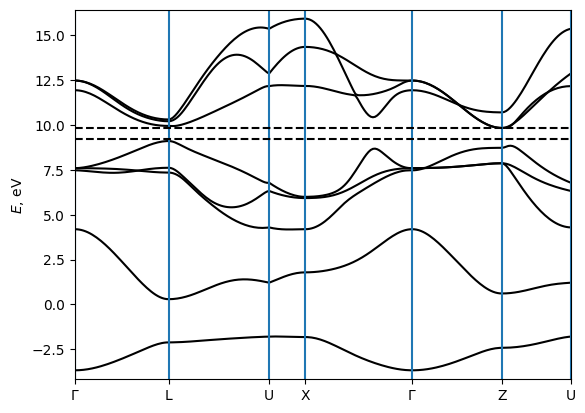We compute the BCD tensor for the hole-doped case. (The electron-doped case is much harder to converge due to band crossings near the CBM.)

Try to calculate BCD for various grids and compare the convergence of the two methods. (NK=120 will be enough to see convergence.)

:

data_GeTe_fsurf_all = {}
data_GeTe_fsea_all = {}

:

from wannierberri import calculators as calc
from wannierberri.smoother import FermiDiracSmoother

efermi = np.linspace(e_vbm_GeTe - 1.5, e_cbm_GeTe, 301, True)
smoother = FermiDiracSmoother(efermi, 300.0)

kwargs = dict(
Efermi=efermi,
smoother=smoother,
)

calculators = {
'berry_dipole_fermi_sea': calc.static.BerryDipole_FermiSea(**kwargs),
'berry_dipole_fermi_surface': calc.static.BerryDipole_FermiSurf(**kwargs),
}

Berry curvature dipole (dimensionless)

| With Fermi sea integral. Eq(29) in Ref <https://www.nature.com/articles/s41524-021-00498-5>_
| Output: :math:D_{\beta\delta} = \int [dk] \partial_\beta \Omega_\delta f

Berry curvature dipole (dimensionless)

| With Fermi surface integral. Eq(8) in Ref <https://journals.aps.org/prl/abstract/10.1103/PhysRevLett.115.216806>_
| Output: :math:D_{\beta\delta} = -\int [dk] v_\beta \Omega_\delta f'


:

for nk in [20, 30, 40, 60, 80, 120]:
grid = wberri.Grid(system_GeTe, NK=nk)
result = wberri.run(
system_GeTe,
grid=grid,
calculators=calculators,
parallel=parallel,
print_Kpoints = False,
)
data_GeTe_fsurf_all[nk] = result.results['berry_dipole_fermi_surface'].dataSmooth
data_GeTe_fsea_all[nk] = result.results['berry_dipole_fermi_sea'].dataSmooth

determining grids from NK=80 (<class 'int'>), NKdiv=None (<class 'NoneType'>), NKFFT=None (<class 'NoneType'>)
Minimal symmetric FFT grid :  [3 3 3]
The grids were set to NKdiv=[20 20 20], NKFFT=[4 4 4], NKtot=[80 80 80]
Grid is regular
The set of k points is a Grid() with NKdiv=[20 20 20], NKFFT=[4 4 4], NKtot=[80 80 80]
generating K_list
Done in 0.18623900413513184 s
excluding symmetry-equivalent K-points from initial grid
Done in 1.052736520767212 s
Done in 1.0575153827667236 s
K_list contains 781 Irreducible points(9.76%) out of initial 20x20x20=8000 grid
Done, sum of weights:0.999999999999985
symgroup : <wannierberri.symmetry.Group object at 0x7f535ed4b640>
processing 781 K points : using  1 processes.
# K-points calculated  Wall time (sec)  Est. remaining (sec)
78             27.0                 242.9
156             48.1                 192.7
234             70.9                 165.6
312             99.5                 149.6
390            121.4                 121.7
468            141.7                  94.8
546            162.2                  69.8
624            182.4                  45.9
702            204.4                  23.0
780            224.4                   0.3
time for processing    781 K-points on   1 processes:   240.9678 ; per K-point          0.3085 ; proc-sec per K-point          0.3085
time1 =  1.158562183380127
Totally processed 781 K-points

:

nk = 20
print("Shape of data: ", data_GeTe_fsurf_all[nk].shape)

print("Maximum value for each components (Fermi surface): ")
print(np.amax(abs(data_GeTe_fsurf_all[nk]), axis=0))

print("Maximum value for each components (Fermi sea): ")
print(np.amax(abs(data_GeTe_fsea_all[nk]), axis=0))

Shape of data:  (301, 3, 3)
Maximum value for each components (Fermi surface):
[[2.75821033e-18 1.78581033e-01 5.36068541e-15]
[1.78581033e-01 9.57931302e-18 1.09150709e-17]
[5.36012901e-15 1.09047721e-17 6.93608374e-18]]
Maximum value for each components (Fermi sea):
[[4.05362734e-19 5.76069248e-02 1.72783080e-15]
[5.76069248e-02 3.37626401e-18 1.77990429e-18]
[1.72920817e-15 1.38700899e-18 8.71348106e-19]]

:

ic = 0
fig, axes = plt.subplots(1, 2, figsize=(12, 4))
for nk in sorted(list(data_GeTe_fsurf_all.keys())):
c = f"C{ic}"
axes.plot(efermi, data_GeTe_fsurf_all[nk][:, 0, 1], c=c, label=f"NK={nk}")
axes.plot(efermi, data_GeTe_fsea_all[nk][:, 0, 1], c=c, label=f"NK={nk}")
ic += 1

axes.set_title("Fermi surface")
axes.set_title("Fermi sea")

for ax in axes:
ax.axhline(0, c="k", lw=1)
ax.axvline(e_vbm_GeTe, c="k", lw=1, ls="--")
ax.axvline(e_cbm_GeTe, c="k", lw=1, ls="--")
ax.set_ylim([-0.05, 0.05])
ax.set_xlabel("Fermi level (eV)")
ax.set_ylabel("Berry curvature dipole")

axes.legend()
plt.tight_layout()
plt.show()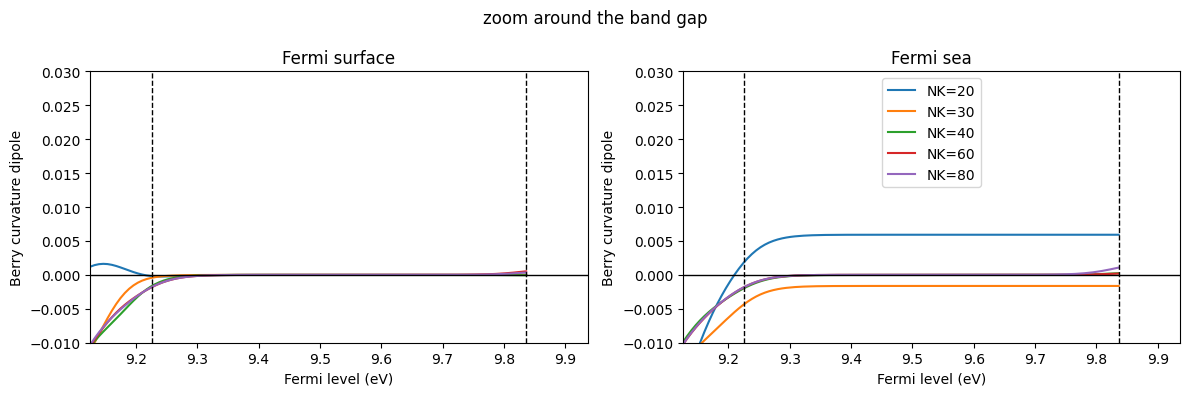Let us focus on the undoped case: Fermi level between the VBM and the CBM. Then, $:nbsphinx-math:frac{partial}{partial k^a} f_0 (\varepsilon_{n:nbsphinx-math:mathbf{k}}) =0$ holds so that the BCD is always 0. For the Fermi surface method, the integrand is 0 at every k point, so the integral is also 0. However, for the Fermi sea method, the integrand is not 0, and the integral becomes 0 only due to a calculation.

:

ic = 0
fig, axes = plt.subplots(1, 2, figsize=(12, 4))
for nk in sorted(list(data_GeTe_fsurf_all.keys())):
c = f"C{ic}"
axes.plot(efermi, data_GeTe_fsurf_all[nk][:, 0, 1], c=c, label=f"NK={nk}")
axes.plot(efermi, data_GeTe_fsea_all[nk][:, 0, 1], c=c, label=f"NK={nk}")
ic += 1

axes.set_title("Fermi surface")
axes.set_title("Fermi sea")

for ax in axes:
ax.axhline(0, c="k", lw=1)
ax.axvline(e_vbm_GeTe, c="k", lw=1, ls="--")
ax.axvline(e_cbm_GeTe, c="k", lw=1, ls="--")
ax.set_xlabel("Fermi level (eV)")
ax.set_ylabel("Berry curvature dipole")
ax.set_ylim(np.array([-0.01, 0.03]) * 1)
ax.set_xlim([e_vbm_GeTe - 0.1, e_cbm_GeTe + 0.1])

axes.legend()
fig.suptitle("zoom around the band gap")
plt.tight_layout()
plt.show():

# Choose the Fermi level index at the middle of the band gap.
ifermi = np.argmin(np.abs(efermi - (e_vbm_GeTe + e_cbm_GeTe) / 2))

nklist = sorted(list(data_GeTe_fsurf_all.keys()))

print("D_xy for the undoped case")
print("NK : ", nklist)
print("Fermi sea: ", [data_GeTe_fsea_all[nk][ifermi, 0, 1] for nk in nklist])
print("Fermi surface: ", [data_GeTe_fsurf_all[nk][ifermi, 0, 1] for nk in nklist])

plt.plot(nklist, [abs(data_GeTe_fsurf_all[NK][ifermi, 0, 1]) for NK in nklist], "o-")
plt.plot(nklist, [abs(data_GeTe_fsea_all[NK][ifermi, 0, 1]) for NK in nklist], "o-")
# plt.yscale("log") # Also try logscale
plt.xlabel("Nk")
plt.ylabel("BCD for the undoped case")
plt.show()

D_xy for the undoped case
NK :  [20, 30, 40, 60, 80, 120]
Fermi sea:  [0.005090029617682658, -0.001636927143447324, -7.100518562204471e-06, -3.727637728706892e-07, 5.031723634526716e-09, 1.0901526500907337e-12]
Fermi surface:  [0.0, 0.0, 0.0, 0.0, 0.0, 0.0]## Further questions

If you are interested, try to answer the following questions: - What happens if one uses an un-symmetrized model? Does Fermi sea BCD with the Fermi energy inside the gap converge to 0? - What happens if one uses the tetrahedron method? - What happens for the zero-temperature case (you may use .data instead of .dataSmooth)?